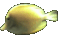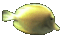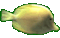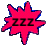Integers & Order of Operations Help     Fun Game Tips:

- Adding positive and negative integers can be compared to winning and losing money.
- A win of \$4 added to a loss of \$6 results in a total loss of \$2. That is (+4)+(-6)=(-2).

- To subtract an integer, you can add its opposite.
- That means (-3)-(+7) is the same as (-3)+(-7) = -10.
- Also (6)-(-2) is the same as (+6)+(+2)=8, because removing a \$2 loss gives a \$2 gain.

- When multiplying 2 integers having the same sign, the result is positive. Example (-4)(-5)= 20.
- When multiplying 2 integers having a different sign, the result is negative. Example (-3)(+6)= -18.
- The positive and negative sign rules for integer division are the same as for integer multiplication.

- The order of operations requires that bracket operations be done before the 4 basic operations +-*/.
- As a general rule, when several of the 4 basic operations occur in succession,
do multiplication and division first (from left to right)
followed by addition and subtraction (from left to right).

- Your Game Score is reduced by the number of fish hits.

- To slow the game speed repeat tap/click on the word Slider.
- To increase the game speed repeat tap/click on the word Math.
- Speed can also be adjusted with a keyboard's - and + keys.

- Refresh/Reload the web page to restart the game.